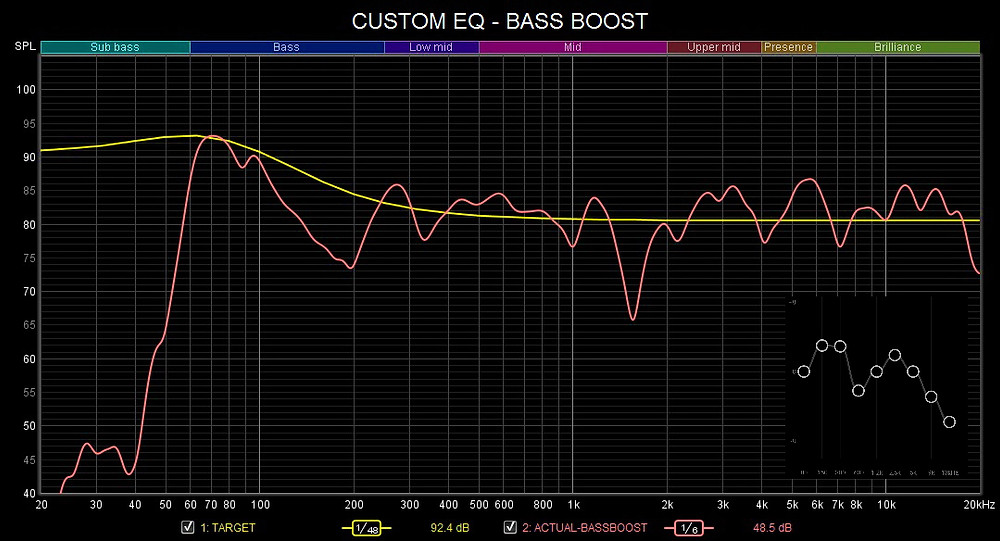Search

# Anker Soundcore 3 Custom EQ - Rock, Harman, Flat, Bass Boost, Pop

Updated: Mar 5

Version 00.31## PRESET

Frequency Response of BassUp On and Off## ROCKFreq. (Hz) /Gain (dB)

80 = -3

150 = +1

300 = +0

600 = -6

1200 = +0

2500 = +2

5000 = +0

9000 = +0

13000 = -3

## HARMAN TARGET CURVE

Updated: March 6, 2022Freq. (Hz) /Gain (dB)

80 = +0

150 = +0

300 = +3

600 = +0

1200 = +0

2500 = +2

5000 = +0

9000 = -3

13000 = -6

## FLATFreq. (Hz) /Gain (dB)

80 = -8

150 = +3

300 = +0

600 = -2

1200 = +0

2500 = +2

5000 = +0

9000 = -4

13000 = -6

## BASS BOOSTFreq. (Hz) /Gain (dB)

80 = +0

150 = +3

300 = +3

500 = -2

700 = +0

1000 = +2

5000 = +0

8000 = -3

12000 = -6

More EQ settings coming soon.